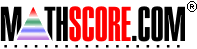Math Practice Online > free > lessons > Florida > 6th grade > Linear Equations

## Linear Equations

Given a linear equation, determine y values given x values. You may need pencil and paper.

 Sample Problems for Linear Equations Lesson for Linear Equations

### This topic aligns to the following state standards

Grade 8: Geo 1. Given an equation or its graph, finds ordered-pair solutions (for example, y = 2x).
Grade 6: Alg 2. Substitutes values for variables in expressions and describes the results or patterns observed.
Grade 8: Alg 6. Evaluates algebraic expressions, equations, and inequalities by substituting integral values for variables and simplifying the results.
Grade 6: Alg 4. Uses pictures, models, manipulatives or other strategies to solve simple one-step linear equations with rational solutions.
Grade 7: Alg 4. Uses pictures, models, manipulatives or other strategies to solve one-step and simple multi- step linear equations.
Grade 8: Alg 3. Solves single- and multiple-step linear equations and inequalities in concrete or abstract form.

Copyright Accurate Learning Systems Corporation 2008.